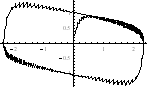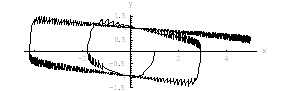János Karsai Models of Impulsive Phenomena Mathematica experiments    Typotex Publishing Company, Budapest 2002   Gallery

 Chapter 1.3 Visualization of the path of motions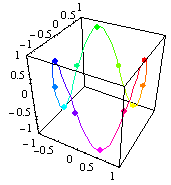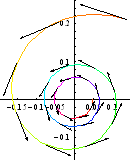Chapter 4.3 Modeling scheme for 1D systems

Direction field, impulse field, and solutions of a 1D system: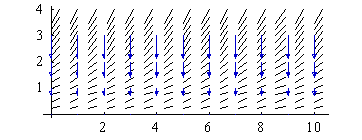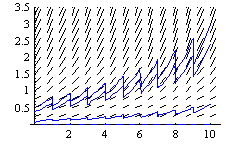Chapter 4.4 Modeling scheme for 2D systems

Impulse field and trajectories of a 2D system: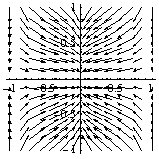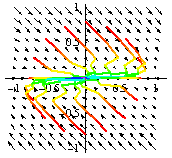Chapter 5.4 Visualization of general impulses

Transformations by 1D and 2D  and impulses: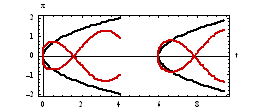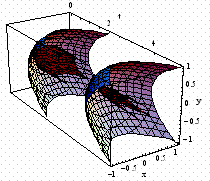Chapter 6.2 Phasemaps of  differential equatios

Phasemaps of a linear system: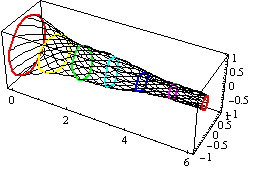Chapter 6.3  Phasemaps of  impulsive systems

Phasemaps of a nonlinear system: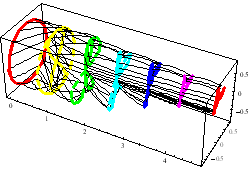Chapter 7.1  Linear systems

A system with singular impulses: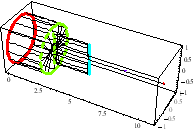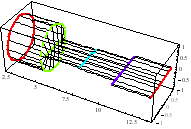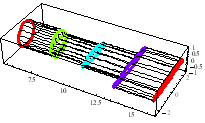Chapter 8.3  Stability by linear approximation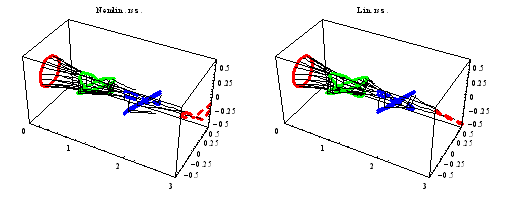Chapter 10.3  Experiments for the Liapunov’s method

Direction and impulse fields and contour lines of the Liapunov’s function: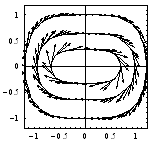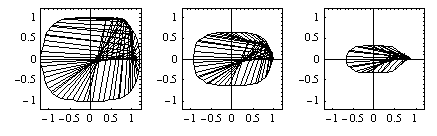Integral curves: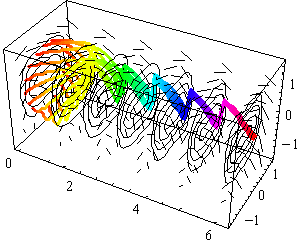Chapter 13.4  Impulsive external force in oscillators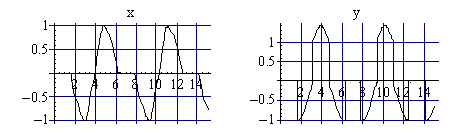Chapter 13.5  Impulsive relaxations oscillations